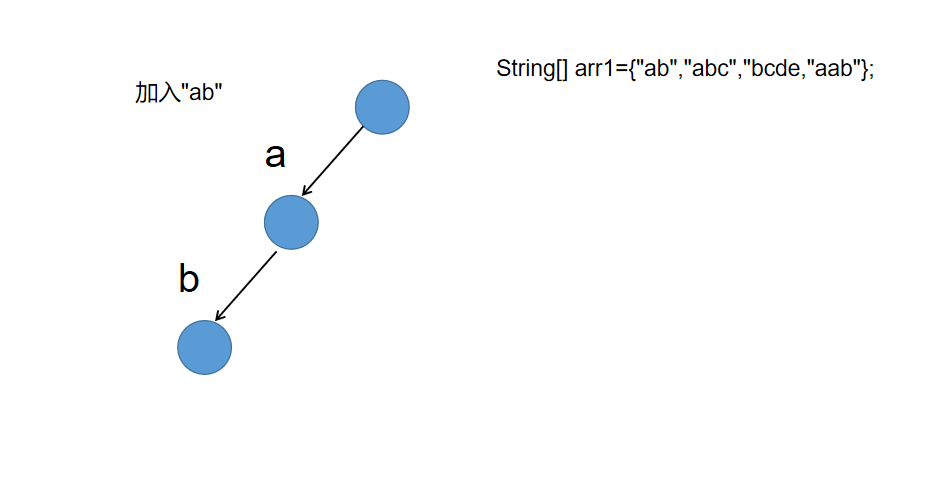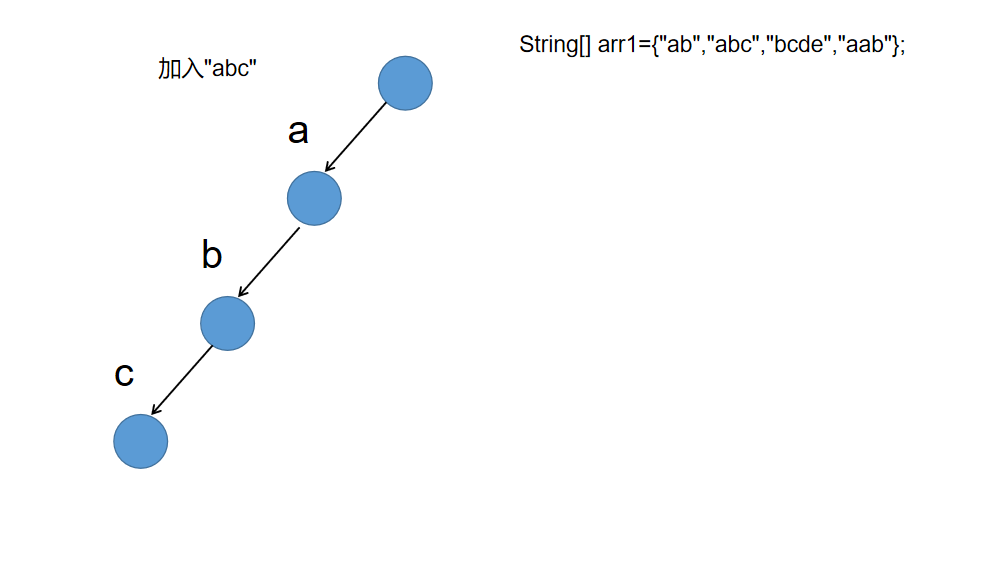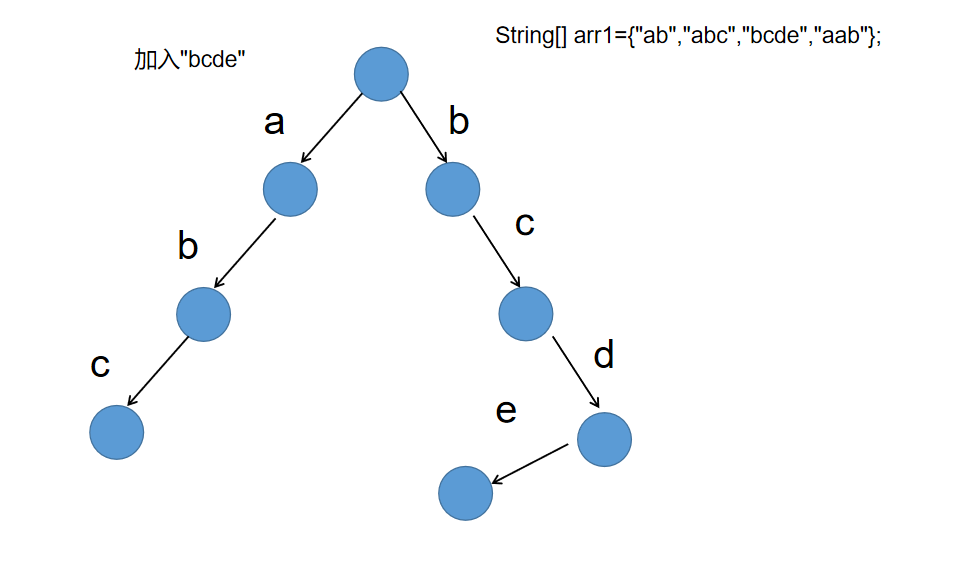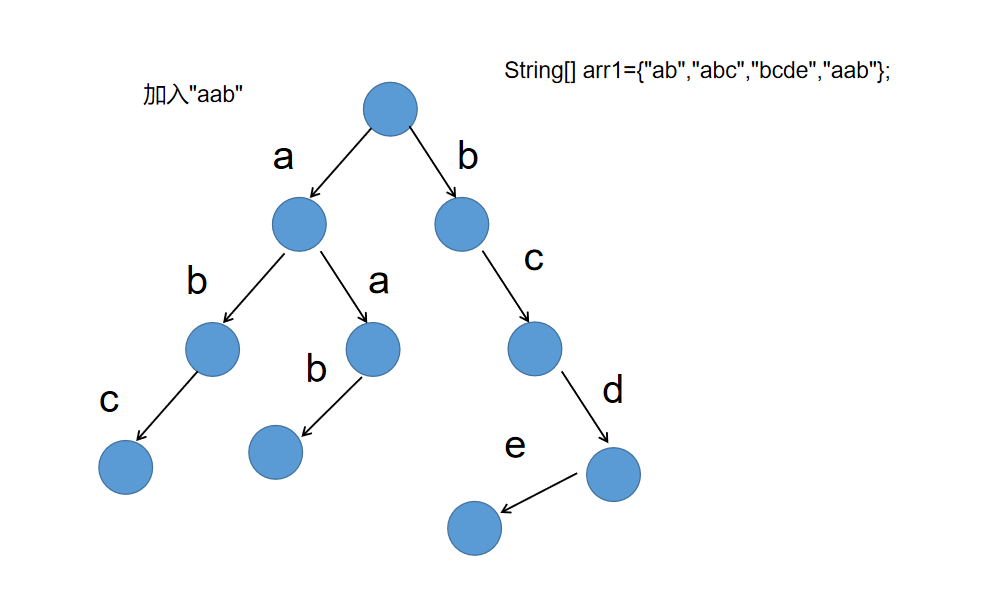• 前缀树

2021-02-06 14:54:39
什么是前缀树 前缀树又叫单词查找树，Tire树，是哈希树的变种。典型应用于统计，排序和保存大量字符串,经常被搜索引擎系统用于文本词频统计。 前缀树的每一个节点会有多个子节点，通往不同子节点的路径上有着不同的...

什么是前缀树

• 前缀树又叫单词查找树，Tire树，是哈希树的变种。典型应用于统计，排序和保存大量字符串,经常被搜索引擎系统用于文本词频统计。
• 前缀树的每一个节点会有多个子节点，通往不同子节点的路径上有着不同的字符串（不仅限于字符串）。
• 优点：可以利用字符串的公共前缀来减少查询时间，最大限度地减少不必要的字符串比较，查询效率比哈希树高。

问题举例
一个字符串类型的数组arr1,另一个字符串类型数组arr2。
（1）arr2中有哪些字符串，是arr1中出现的?请打印。
（2）arr2中有哪些字符串，是作为arr1中某个字符串前缀出现的?请打印。
（3）arr2中有哪些字符串，是作为arr1中某个字符串前缀出现的?请打印arr2中出现次数最大的前缀。
构造前缀树
下面以数组String[] arr1={“ab”,“abc”,“bcde”,“aab”}; 为例构造一个前缀树

1.加入"ab"2.加入"abc"3.加入"bcde"4.加入"aab"前缀树的代码实现

public class TrieDemo {
public static void main(String[] args) {
String[] arr1={"ab","abc","bcde"};
TrieTree trieTree = new TrieTree();
trieTree.delete("abc");
int num = trieTree.search("abc");
System.out.println("num="+num);
int prefixNumber = trieTree.prefixNumber("abc");
System.out.println("prefixNumber="+prefixNumber);
}
//先构造一个类表示前缀树的结点
public static class TrieNode {
int path;  //path表示经过这个结点的字符串个数(这个值不包含以这个结点为终点的字符串)
int end;   //end表示在这个结点结束的字符串的个数
TrieNode[] nexts;

public TrieNode() {
this.path = 0;
this.end = 0;
nexts = new TrieNode;
}

}

//构造一个类表示前缀树
public static class TrieTree {
TrieNode root;

public TrieTree() {
//初始化根结点
this.root = new TrieNode();
}

//1.加入字符串的方法
char[] chars = str.toCharArray();
TrieNode node = root;
int index = 0;
for (int i = 0; i < chars.length; i++) {
index = chars[i] - 'a';
if (node.nexts[index] == null) { //说明没有这个结点
//创建一个新的结点
TrieNode trieNode = new TrieNode();
//让当前结点的nexts对应的结点指向这个新的结点
node.nexts[index] = trieNode;
}
//往下走,当前的node变成下一个node
node = node.nexts[index];
//把这个node的path+1
node.path++;
}
node.end++;
}
//2.查询前缀树中有几个这样的字符串
public int search(String str) {
if(str==null||str==""){
return 0;
}
char[] chars = str.toCharArray();
int index = 0;
TrieNode node = root;
for (int i = 0; i < chars.length; i++) {
index = chars[i] - 'a';
//如果找查找过程中node.nexts[index]为空表示chars还没有遍历完,说明树中没有这个字符串
if (node.nexts[index] == null) {
return 0;
}
node = node.nexts[index];
}
return node.end;
}
//3.删除字符串的方法
public void delete(String str) {
//1.先查询树中是否有这样一个字符串
//说明树中没有这样一个字符串
if (search(str) == 0) {
return;
}
TrieNode node = root;
char[] chars = str.toCharArray();
int index = 0;
for (int i = 0; i < chars.length; i++) {
index = chars[i] - 'a';
if (node.nexts[index].path == 0) { //这个时候说明node.nexts[index]这个结点上已经没有数据了,删除这个结点
node.nexts[index] = null;
return;
}
node = node.nexts[index];
node.path--;
}
node.end--;
}

//4.查询前缀树中有多少个以prefix为前缀的字符串
public int prefixNumber(String prefix) {
if (prefix == "" || prefix == null) {
return 0;
}
char[] chars = prefix.toCharArray();
int index = 0;
TrieNode node = root;
for (int i = 0; i < chars.length; i++) {
index = chars[i] - 'a';
if (node.nexts[index] == null) {
return 0;
}
node = node.nexts[index];
}
return node.path;
}
}

}

展开全文数据结构 算法
• 路由前缀树 go语言中gin框架的路由实现就是利用前缀树，可以看看它的源代码是如何实现的。 考虑一下，路由或者说路径的特点，是以 / 分隔的单词组成的，那我们将 / 的每一部分挂靠在前缀树上就可以了。如下图所示：...

路由功能是web框架中一个很重要的功能，它将不同的请求转发给不同的函数(handler)处理，很容易能想到，我们可以用一个字典保存它们之间的对应关系，字典的key存放path，value存放handler。当一个请求过来后，使用 routers.get(path, None) 就可以找到对应的handler。

利用字典实现路由可以参考我的这篇文章：动手实现web框架 。

使用字典有一个问题，不支持动态路由。如果路由像这样呢？

/hello/:name/profile

name前面是通配符: ，表示这是个动态的值。一个解决办法是使用前缀树trie。

前缀树

leetcode中有这个算法，点这里 查看。

前缀树前缀树，首先是一棵树。不同的是树中一个节点的所有子孙都有相同的前缀。前缀树将单词中的每个字母依次插入树中，插入前首先确认该单词是否存在，不存在才创建新节点，如果一个单词已经全部插入，则将末尾单词设置为标志位。

type Node struct {

isWord bool // 是否是单词结尾

next map[string]*Node // 子节点

}

type Trie struct {

root *Node

}

以单词leetcode，leetd和code为例，首先一次插入leetcode中的每个单词，然后插入leetd的时候，leet在树中已经存在，跳过往下，现在要插入字母d，不存在，所以新建节点插入树中，并将该节点的isWord置位true，表明到了单词末尾。

最终插入结果为：func (this *Trie) Insert(word string) {

cur := this.root

for _, w := range []rune(word) {

c := string(w)

if cur.next[c] == nil {

cur.next[c] = &Node{next: make(map[string]*Node)}

}

cur = cur.next[c]

}

cur.isWord = true

}

那么，当我们要搜索单词leetd的时候，从根节点开始查找，如果找到某条路径是leetd，并且末尾的d是单词标志位，则表示搜索成功。

func (this *Trie) Search(word string) bool {

cur := this.root

for _, w := range []rune(word) {

c := string(w)

if cur.next[c] == nil {

return false

}

cur = cur.next[c]

}

return cur.isWord

}

明白了前缀树的原理，我们来看看路由匹配是如何利用前缀树来实现的。

路由前缀树

go语言中gin框架的路由实现就是利用前缀树，可以看看它的源代码是如何实现的。

考虑一下，路由或者说路径的特点，是以 / 分隔的单词组成的，那我们将 / 的每一部分挂靠在前缀树上就可以了。如下图所示：

还有一点需要考虑，我们在用web框架定义路由的时候，常见的做法是根据不同的HTTP方法来定义。比如：

// 以go语言gin框架为例

g := gin.New()

g.GET("/hello", Hello)

g.POST("/form", Form)

对于同一个路径，可能有多个方法支持。所以我们要以不同HTTP方法为树根创建前缀树。当一个GET请求过来的时候，就从GET树上搜索，POST请求就从POST树上搜索。除了为不同的HTTP方法定义树之外，还要给那些是通配符的节点增加一个标志位。所以，我们的路由前缀树结构看起来像这样：

type node struct {

path string // 路由路径

part string // 路由中由'/'分隔的部分

children map[string]*node // 子节点

isWild bool // 是否是通配符节点

}

type router struct {

root map[string]*node // 路由树根节点

route map[string]HandlerFunc // 路由处理handler

}

依照上面的前缀树算法的实现，照葫芦画瓢，我们可以写出插入路由和搜索路由的方法：

func (r *router) addRoute(method, path string, handler HandlerFunc) {

parts := parsePath(path)

if _, ok := r.root[method]; !ok {

r.root[method] = &node{children: make(map[string]*node)}

}

root := r.root[method]

key := method + "-" + path

// 将parts插入到路由树

for _, part := range parts {

if root.children[part] == nil {

root.children[part] = &node{

part: part,

children: make(map[string]*node),

isWild: part == ':' || part == '*'}

}

root = root.children[part]

}

root.path = path

// 绑定路由和handler

r.route[key] = handler

}

// getRoute 获取路由树节点以及路由变量

func (r *router) getRoute(method, path string) (node *node, params map[string]string) {

params = map[string]string{}

searchParts := parsePath(path)

// get method trie

var ok bool

if node, ok = r.root[method]; !ok {

return nil, nil

}

// 在该方法的路由树上查找该路径

for i, part := range searchParts {

var temp string

// 查找child是否等于part

for _, child := range node.children {

if child.part == part || child.isWild {

// 添加参数

if child.part == '*' {

params[child.part[1:]] = strings.Join(searchParts[i:], "/")

}

if child.part == ':' {

params[child.part[1:]] = part

}

temp = child.part

}

}

// 遇到通配符*，直接返回

if temp == '*' {

return node.children[temp], params

}

node = node.children[temp]

}

return

}

上面的代码是我自己实现的一个web框架 gaga 中路由前缀树相关的代码，有需要的可以去看看源代码。另外，欢迎star 呀。

其中的 addRoute 用来将路由插入到对应method的路由树中，如果节点是通配符，将其设置为 isWild ， 同时绑定路由和handler方法。

getRoute 方法首先查找路由方法对应的路由前缀树，然后在树中查找是否存在该路径。

总结

前缀树trie算法不光可以用在路由的实现上，搜索引擎中自动补全的实现，拼写检查等等都是用trie实现的。trie树查找的时间和空间复杂度都是线性的，效率很高，很适合路由这种场景使用。

路由的实现上，go语言中 httpRouter 这个库除了使用前缀树之外，还加入了优先级，有兴趣的可以看看它的源码了解下。

有疑问加站长微信联系(非本文作者)展开全文Mysql前缀树
• 为什么要有前缀树 查询一个字符串是否在一个字符串数组出现多少次，可以使用哈希表保存字符串。 但是如果想要查询以he开头的字符串有多少个的时候，哈希表是不支持这样的查询的。 如果使用暴力方法，直接对字符串...

为什么要有前缀树

查询一个字符串是否在一个字符串数组出现多少次，可以使用哈希表保存字符串。
但是如果想要查询以he开头的字符串有多少个的时候，哈希表是不支持这样的查询的。
如果使用暴力方法，直接对字符串数组中每个字符串进行遍历，时间复杂度会达到 O ( N ∗ K ) O(N * K) ，K为前缀长度。看似这个还是可以接受的，毕竟 O ( N ) O(N) 的时间复杂度本身已经挺小的，但是前缀树可以让时间复杂度接近常数 O ( K ) O(K)
那么下面就介绍一下什么是前缀树，如何建立（插入）前缀树，如何查询某个字符串有多少个删除某个字符串，以及查询有多少个字符串是以某个前缀开头的。

什么是前缀树？

先看图来个直观感受如何完成上面前缀树的建立（插入）过程？

1. 单个字符串中，字符从前到后的加到同一颗多叉树上。
2. 字符放在树干上（不是节点），节点上有专属的数据项（常见的是pass和end值）
3. 所有样本都这样添加，如果没有路就新建，如果有路就复用。
4. 沿途节点的pass值增加1，每个字符串结束时来到的节点end值增加1.

建立前缀树

最开始使用，根节点开始，节点不代表任何字符，而是使用连接节点的树枝代表字符，一个分支代表一个字母，本次demo仅仅支持26个字母，所以使用长度为26的数组就可以。如果想要支持所有字符，可以使用哈希表实现（key=字符，value=Node引用）。
每个节点记录通过该节点的单词数目，以该节点结束的单词数目，以及该节点到下一个节点的路径（路径就是代表一个字符）。

class Node {
int pass = 0;
int end = 0;
Node[] paths = new Node;
}

按照上面描述的插入过程，完成如下代码
比较容易忘记的就是上来就要对root.pass++，并且要记得是在树枝上记录字符，而不是节点，因此在操作下一个节点的时候，这时候就要更新next.pass++
最后单词最后一个字符处理结束后，退出循环，这时候curNode指向仍是最后一个字符树枝指向的节点，这时候要表示字符串结束，需要curNode.end++

public void insertTries(String word) {
if (word == null) {
return;
}
char[] str = word.toCharArray();
Node node = root;
node.pass++;
int index = 0;
for (int i = 0; i < str.length; i++) {
index = str[i] - 'a';
if (node.paths[index] == null) {
node.paths[index] = new Node();
}
node = node.paths[index];
node.pass++;
}
node.end++;
}

int search(String str)查询某个字符串在结构中有几个

public int searchTries(String str) {
if (str == null)  {
return 0;
}
Node node = root;

char[] chars = str.toCharArray();
int index;
for (int i = 0; i < chars.length; i++) {
index = chars[i] - 'a';
Node next = node.paths[index];
if (next == null) {
return 0;
}
node = next;
}
return node.end;
}

删除指定单词

1. 必须要先搜索单词是否在前缀树，否则可能删除到后面发现实际上没有这个单词，例如只有abc，但是要删除abcd，删除到abc发现没有d，但是却没有办法恢复原来的情况。
2. 在Java中可以对node.pass == 0情况优化，直接把节点设置为null，JVM会自动回收后面的节点。
public void delete(String word) {
if (search(word) != 0) {
Node node = root;
node.pass--;
int index = 0;
char[] chars = word.toCharArray();
for (int i = 0; i < chars.length; i++) {
index = chars[i] - 'a';
if (--node.paths[index].pass == 0) {
node.paths[index] = null
return;
}
node = node.paths[index];
}
node.end--;
}

}

以某个前缀开头的单词有多少个

1. 字母前缀就是前缀树节点的pass属性，有多少个单词通过该节点，pass就是多少
public int prefixWithStr(String prefix) {
if (prefix == null) {
return 0;
}
Node node = root;
int index;
char[] chars = prefix.toCharArray();
for (int i = 0; i < chars.length; i++) {
index = chars[i] - 'a';
Node next = node.paths[index];
if (next == null) {
return 0;
}
node = next;
}

return node.pass;

拓展：使用HashMap实现全字符的前缀树

package class05;

import java.util.HashMap;

/**
* @author mingyan wang
* @date 2021/1/20 4:40 PM
*/
public class generalTries {
Node root = new Node();
class Node {
int pass = 0;
int end = 0;
HashMap<Integer, Node> paths = new HashMap<>();
}

public void insert(String word) {
if (word == null) {
return;
}
Node node = root;
node.pass++;
char[] chars = word.toCharArray();
int index = 0;
for (int i = 0; i < chars.length; i++) {
index = (int) chars[i];
if (!node.paths.containsKey(index)) {
node.paths.put(index, new Node());
}
node = node.paths.get(index);
node.pass++;
}
node.end++;
}

public int search(String word) {
if (word == null) {
return 0;
}
Node node = root;
char[] chars = word.toCharArray();
int index;
for (int i = 0; i < chars.length; i++) {
index = (int) chars[i];
if (!node.paths.containsKey(index)) {
return 0;
}
node = node.paths.get(index);
}
return node.end;
}

public int prefixWith(String prefix) {
if (prefix == null) {
return 0;
}
Node node = root;
char[] chars = prefix.toCharArray();
int index = 0;
for (int i = 0; i < chars.length; i++) {
index = chars[i];
if (!node.paths.containsKey(index)) {
return 0;
}
node = node.paths.get(index);
}
return node.pass;

}

public void delete(String word) {
if (search(word) != 0) {
Node node = root;
node.pass--; // 很容易忘记
char[] chars = word.toCharArray();
int index;
for (int i = 0; i < chars.length; i++) {
index = chars[i];
Node next = node.paths.get(index);
if (--next.pass == 0) {
node.paths.remove(index);
return;
}
node = next;
}
node.end--;
}
}

}

拓展：Trie对数器

对数器采用的方式是实现一个HashMap，在该Map中存储字符串和字符串出现的次数。最容易错的地方就在统计前缀出现次数，应该是count +=而不是count++

package class05;

import java.util.HashMap;

/**
* @author mingyan wang
* @date 2021/1/20 5:01 PM
*/
public class RightTrie {
private HashMap<String, Integer> box;

public RightTrie() {
box = new HashMap<>();
}

public void insert(String word) {
if (!box.containsKey(word)) {
box.put(word, 1);
} else {
box.put(word, box.get(word) + 1);
}
}

public int search(String word) {
if (box.containsKey(word)) {
return box.get(word);
}
return 0;
}

public void delete(String word) {
if (box.containsKey(word)) {
if (box.get(word) == 1) {
box.remove(word);
} else {
box.put(word, box.get(word) - 1);
}
}
}

public int prefixWith(String prefix) {
int count = 0;
for (String word: box.keySet()) {
if (word.startsWith(prefix)) {
count += box.get(word);
}
}
return count;
}
// for test
public static String generateRandomString(int strLen) {
char[] ans = new char[(int) (Math.random() * strLen) + 1];
for (int i = 0; i < ans.length; i++) {
int value = (int) (Math.random() * 6);
ans[i] = (char) (97 + value);
}
return String.valueOf(ans);
}

// for test
public static String[] generateRandomStringArray(int arrLen, int strLen) {
String[] ans = new String[(int) (Math.random() * arrLen) + 1];
for (int i = 0; i < ans.length; i++) {
ans[i] = generateRandomString(strLen);
}
return ans;
}

public static void main(String[] args) {
int arrLen = 100;
int strLen = 20;
int testTimes = 100000;
for (int i = 0; i < testTimes; i++) {
String[] arr = generateRandomStringArray(arrLen, strLen);
Tries.Trie1 trie1 = new Tries.Trie1();
generalTries trie2 = new generalTries();
RightTrie right = new RightTrie();
for (int j = 0; j < arr.length; j++) {
double decide = Math.random();
if (decide < 0.25) {
trie1.insert(arr[j]);
trie2.insert(arr[j]);
right.insert(arr[j]);
} else if (decide < 0.5) {
trie1.delete(arr[j]);
trie2.delete(arr[j]);
right.delete(arr[j]);
} else if (decide < 0.75) {
int ans1 = trie1.search(arr[j]);
int ans2 = trie2.search(arr[j]);
int ans3 = right.search(arr[j]);
if (ans1 != ans2 || ans2 != ans3) {
System.out.println("Oops!");
}
} else {
int ans1 = trie1.prefixNumber(arr[j]);
int ans2 = trie2.prefixWith(arr[j]);
int ans3 = right.prefixWith(arr[j]);
if (ans1 != ans3 ) {
System.out.println("Ans 1 != Right");
System.out.println("ANS1" + ans1);
System.out.println("ANS3" + ans3);
System.out.println("ANS2" + ans2);
}
if (ans2 != ans3 ) {
System.out.println("Ans 2 != Right");
}
if (ans1 != ans2 || ans2 != ans3) {
System.out.println("Oops!");
}
}
}
}
System.out.println("finish!");

}
}

以上内容参考自左程云老师授课内容，感谢左程云老师，详细讲解请听左程云老师最新课程，目前左程云老师在腾讯课堂马士兵老师教育下授课。

展开全文算法 数据结构
• 上篇内容有在介绍 Gin 的路由实现时提到了前缀树，这次我们稍微深入探究一下前缀树的实现。本文以一道编程题为例，讲述前缀树的实现，以及前缀树的一种优化形态压缩前缀树。MapSum 问题LeetCode 上有一道编程题是...

上篇内容有在介绍 Gin 的路由实现时提到了前缀树，这次我们稍微深入探究一下前缀树的实现。

本文以一道编程题为例，讲述前缀树的实现，以及前缀树的一种优化形态压缩前缀树。

MapSum 问题

LeetCode 上有一道编程题是这样的

实现一个 MapSum 类里的两个方法，insert 和 sum。

对于方法 insert，你将得到一对(字符串，整数)的键值对。字符串表示键，整数表示值。如果键已经存在，那么原来的键值对将被替代成新的键值对。

对于方法 sum，你将得到一个表示前缀的字符串，你需要返回所有以该前缀开头的键的值的总和。

示例 1:

输入: insert("apple", 3), 输出: Null

输入: sum("ap"), 输出: 3

输入: insert("app", 2), 输出: Null

输入: sum("ap"), 输出: 5

前缀树

根据题意，我们定义的 MapSum 的数据结构为：

type MapSum struct {

char byte

children map[byte]*MapSum

val int

}

/** Initialize your data structure here. */

func Constructor() MapSum {

}

func (this *MapSum) Insert(key string, val int) {

}

func (this *MapSum) Sum(prefix string) int {

}

假设输入数据为：

m := Constructor()

m.Insert("inter", 1)

m.Insert("inner", 2)

m.Insert("in", 2)

m.Insert("if", 4)

m.Insert("game", 8)

则构造的前缀树应该是：前缀树特性：

根节点不包含字符，除根节点外的每一个子节点都包含一个字符

从根节点到某一节点的路径上的字符连接起来，就是该节点对应的字符串。

每个节点的所有子节点包含的字符都不相同。

Insert 函数

Insert 函数的签名：

func (this *MapSum) Insert(key string, val int)

我们把 this 当做父节点，当插入的 key 长度为 1 时，则直接说明 key 对应的节点应该是 this 的孩子节点。

if len(key) == 1 {

for i, m := range this.children {

// c 存在与孩子节点

// 直接更新

if i == c {

m.val = val

return

}

}

// 未找到对应孩子

// 直接生成新孩子

this.children[c] = &MapSum{

char: c,

val: val,

children: make(map[byte]*MapSum),

}

return

}

当插入的 key 长度大于 1，则寻找 key 对应的子树，如果不存在，则插入新孩子节点；设置 this = this.children[key] 继续迭代;

c := key

for i, m := range this.children {

if i == c {

key = key[1:]

this = m

continue walk

}

}

// 未找到节点

this.children[c] = &MapSum{

char: c,

val: 0,

children: make(map[byte]*MapSum),

}

this = this.children[c]

key = key[1:]

continue walk

Sum 函数

Sum 函数签名：

func (this *MapSum) Sum(prefix string) int

Sum 函数的基本思想为：先找到前缀 prefix 对应的节点，然后统计以该节点为树根的的子树的 val 和。

// 先找到符合前缀的节点

// 然后统计和

for prefix != "" {

c := prefix

var ok bool

if this, ok = this.children[c]; ok {

prefix = prefix[1:]

continue

} else{

// prefix 不存在

return 0

}

}

return this.sumNode()

sumNode 函数统计了子树的 val 和，使用递归遍历树：

s := this.val

for _, child := range this.children{

s += child.sumNode()

}

return s

以上是一种标准的前缀树的做法。当字符串公用的节点比较少的时候，对于每个字符都要创建单独的节点，有点浪费空间。有一种压缩前缀树的算法，在处理前缀树问题的时候能够使用更少的节点。

压缩前缀树

对与上面的例子来说，压缩前缀树是这样的结果：对于该例子来说，明显少了很多节点。另外，我们的 MapSum 结构体也稍微有了变化：

type MapSum struct {

// 之前的 char byte 变成了 key string

key string

children map[byte]*MapSum

val int

}

Insert

压缩前缀树与前缀树的实现不同点在于节点的分裂。比如，当树中已经存在 "inner", "inter" 的情况加，再加入 "info" 时，原 "in" 节点需要分裂成 "i" -> "n" 两个节点，如图：在 Insert 时，需要判断当前插入字符串 key 与 节点字符串 this.key 的最长公共前缀长度 n：

minLen := min(len(key), len(this.key))

// 找出最长公共前缀长度 n

n := 0

for n < minLen && key[n] == this.key[n] {

n ++

}

然后拿 n 与 len(this.key) 比较，如果比 this.key 长度短，则 this.key 需要分裂，否则，不需要分裂。

this 节点分裂逻辑：

// 最前公共前缀 n < len(this.key)

// 则该节点需要分裂

child := &MapSum{

val: this.val,

key: this.key[n:],

children: this.children,

}

// 更新当前节点

this.key = this.key[:n]

this.val = 0

this.children = make(map[byte]*MapSum)

this.children[child.key] = child

然后再判断 n 与 len(key)，如果 n == len(key)，则说明 key 对应该节点。直接更新 val

if n == len(key) {

this.val = val

return

}

n < len(key) 时，如果有符合条件子树，则继续迭代，否则直接插入孩子节点：

key = key[n:]

c := key

// 如果剩余 子key 的第一个字符存在与 children

// 则继续向下遍历树

if a, ok := this.children[c]; ok {

this = a

continue walk

} else{

// 否则，新建节点

this.children[c] = &MapSum{

key: key,

val: val,

children: make(map[byte]*MapSum),

}

return

}

以上是压缩前缀树的做法。

算法优化

上述 MapSum 的 children 使用的是 map，但是 map 一般占用内存较大。可以使用 节点数组children + 节点前缀数组 indices 的方式维护子节点，其中 indices 与 children 一一对应。

此时的结构体应该是这样的：

type MapSum struct {

key string

indices []byte

children []*MapSum

val int

}

查找子树时，需要拿 key[:n] 与 indices 中的字符比较，找到下标后继续迭代子树；未找到时插入子树即可。

以上。

Y_xx

相关内容：

有疑问加站长微信联系(非本文作者)

展开全文• trie，又称前缀树或字典树，是一种有序树，用于保存关联数组，其中的键通常是字符串。与二叉查找树不同，键不是直接保存在节点中，而是由节点在树中的位置决定。一个节点的所有子孙都有相同的前缀，也就是这个节点...算法 leetcode 树结构 python
• 字典树（前缀树）（Trie）-C++简单实现 文章目录字典树（前缀树）（Trie）-C++简单实现字典树是什么特点优点我自己的一些理解实现（c++）TrieNode：Trie:能力有限，希望本文能对你有些许帮助????。 之前在刷 ...leetcode 算法
• 我们在《字典树（前缀树、Trie树）》这一篇文章中详细介绍了Trie树，本文将利用Python语言实现它。 我们以Trie来命名我们的Trie树类，它实例化后代表了我们Trie树中的每一个节点。每个节点包含以下两个字段： ...算法 数据结构 字典树 前缀树 Trie树
• 手写前缀树

2021-10-29 17:11:37
一篇文章 ** 单词段出现过几次，看完前缀树都有了答案。 思路 1 构建一棵树 需要记录每个节点的 pass 经过数量 end 单词末尾是这个字母的数量 2 node节点上 需要有个一 数组 26 大小 用于存放这个节点的叶子...前缀树 算法
• Trie树，又叫字典树、前缀树（Prefix Tree）、单词查找树 或 键树，是一种多叉树结构。如下图： 从上图可以归纳出Trie树的基本性质： 根节点不包含字符，除根节点外的每一个子节点都包含一个字符。 从根节点到某一...
• 前缀树 LeetCode题目中有关前缀树的描述：Trie前缀树-字典树 前缀树 Trie树，即字典树，又称单词查找树或键树，是一种树形结构，是一种哈希树的变种。典型应用是用于统计和排序大量的字符串（但不仅限于字符串），...
• 前缀树（字典树） 所以也叫 Trie树 – 字典树/单词查找树/键树 ，是一种树形结构，是一种哈希树的变种，典型应用是用于统计，排序和保存大量的字符串（但不仅限于字符串），所以经常被搜索引擎系统用于文本词频...数据结构
• 前缀树的节点声明 public static class TrieNode{ public int pass; public int end; public TrieNode[] nexts; //一个节点连着那些字符 public TrieNode() { pass = 0; end = 0; nexts = new ...算法 java 数据结构
•算法 前缀树 python
• 前缀树 package cn.hq; /** * @Author: hq * @Date: 2021/3/2 16:21 * @Description:实现一个前缀树，包含insert，search和startWith这三个操作 * 前缀树： */ class Node { boolean isEnd = false; // 假设...前缀树 java
• 前缀树讲解

2021-01-09 20:48:46
前缀树 功能：以树的形式存放单词组 例： in inter input apple append 红色表示一个单词的结束 代码 先给出代码，运行了解一下前缀树的用处： #include <stdio.h> #include <string.h> #include <...c语言
• 摘要： 前缀树，字典树，插入查询逻辑，Java实现，时间复杂度分析前缀树介绍Trie树又被称为前缀树、字典树，把单词字母一条一条灌进一棵树中，每个节点是a-z之间的字母，对于都是数字的字符串，字符集就是0-9， 每一...
• Trie [traɪ] 读音和 try 相同，也称字典树，前缀树，单词查找树等。 前缀树是一种树形数据结构，用于高效地存储和检索字符串数据集中的键。这一数据结构有相当多的应用场景，例如自动补完和拼写检查。 Trie的节点 ...数据结构
• Trie（发音类似 “try”）或者说 前缀树 是一种树形数据结构，用于高效地存储和检索字符串数据集中的键。这一数据结构有相当多的应用情景，例如自动补完和拼写检查。 请你实现 Trie 类： Trie() 初始化前缀树对象。...
• 引言 前缀树——trie 树，也叫作“单词查找树”、“字典树”。 它属于多叉树结构，典型应用场景是统计
• 字典树又称单词查找树、前缀树、Trie树等，是一种树形结构，是一种哈希树的变种。典型应用是用于统计，排序和保存大量的字符串（但不仅限于字符串），所以经常被搜索引擎系统用于文本词频统计。它可以利用字符串的...算法 数据结构 字典树 前缀树 Trie树
• 1 #coding=utf-82 #字典嵌套牛逼,别人写的,这样每一层非常多的东西,搜索就快了,高26.所以整体搜索一个不关多大的单词表,还是O(1).34 '''5 Python 字典 setdefault() 函数和get() 方法类似, 如果键不存在于字典中，...
• 在计算机科学中，trie，又称前缀树或字典树，是一种有序树，用于保存关联数组，其中的键通常是字符串。与二叉查找树不同，键不是直接保存在节点中，而是由节点在树中的位置决定。一个节点的所有子孙都有相同的前缀，...
• 前缀树，又叫字典树，trie树。是一种多叉树。 用途 单词补全/预测 拼写检查 9建输入 IP路由查找（最长前缀匹配） 数组中两个树最大异或值 特点 根节点是空字符 每个节点所有子节点都不同 根到叶子，路径上...前缀树 字典树 trie 路由查找
• 前缀树是一种具有特定规则的N叉树，即使在不熟悉前缀树概念的前提下，我们也可以根据题目中给出的条件及图例分析并完成代码前缀树 字典树 trie
• leetcode 前缀树

2021-04-14 14:44:15
Trie [traɪ] 读音和 try 相同，它的另一些名字有：字典树，前缀树，单词查找树等。 介绍 Trie???? Trie 是一颗非典型的多叉树模型，多叉好理解，即每个结点的分支数量可能为多个。 为什么说非典型呢？因为它和一般......